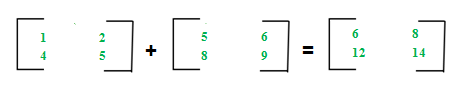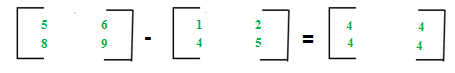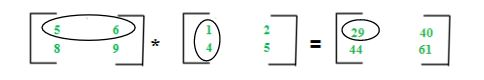# Different Operations on Matrices

For introduction on matrices, you can refer the following article: Matrix Introduction
In this article, we will discuss various operations on matrices and their properties:

The addition of two matrices A m*n and Bm*n gives a matrix Cm*n. The elements of C are sum of corresponding elements in A and B which can be shown as:The algorithm for addition of matrices can be written as:

```for i in 1 to m
for j in 1 to n
cij = aij + bij```

Key points:

• Addition of matrices is commutative which means A+B = B+A
• Addition of matrices is associative which means A+(B+C) = (A+B)+C
• The order of matrices A, B and A+B is always same
• If order of A and B is different, A+B can’t be computed
• The complexity of addition operation is O(m*n) where m*n is order of matrices

Matrices Subtraction –
The subtraction of two matrices Am*n and Bm*n gives a matrix Cm*n. The elements of C are difference of corresponding elements in A and B which can be represented as:The algorithm for subtraction of matrices can be written as:

```for i in 1 to m
for j in 1 to n
cij = aij-bij```

Key points:

• Subtraction of matrices is non-commutative which means A-B ≠ B-A
• Subtraction of matrices is non-associative which means A-(B-C) ≠ (A-B)-C
• The order of matrices A, B and A-B is always same
• If order of A and B is different, A-B can’t be computed
• The complexity of subtraction operation is O(m*n) where m*n is order of matrices

Matrices Multiplication –
The multiplication of two matrices Am*n and Bn*p gives a matrix Cm*p. It means number of columns in A must be equal to number of rows in B to calculate C=A*B. To calculate element c11, multiply elements of 1st row of A with 1st column of B and add them (5*1+6*4) which can be shown as:The algorithm for multiplication of matrices A with order m*n and B with order n*p can be written as:

```for i in 1 to m
for j in 1 to p
cij = 0
for k in 1 to n
cij += aik*bkj```

Key points:

• Multiplication of matrices is non-commutative which means A*B ≠ B*A
• Multiplication of matrices is associative which means A*(B*C) = (A*B)*C
• For computing A*B, the number of columns in A must be equal to number of rows in B
• Existence of A*B does not imply existence of B*A
• The complexity of multiplication operation (A*B) is O(m*n*p) where m*n and n*p are order of A and B respectively
• The order of matrix C computed as A*B is m*p where m*n and n*p are order of A and B respectivelyMy Personal Notes arrow_drop_up

This article is contributed by Sonal Tuteja. If you like GeeksforGeeks and would like to contribute, you can also write an article using contribute.geeksforgeeks.org or mail your article to contribute@geeksforgeeks.org. See your article appearing on the GeeksforGeeks main page and help other Geeks.

Please Improve this article if you find anything incorrect by clicking on the "Improve Article" button below.

Article Tags :
Practice Tags :

5

Please write to us at contribute@geeksforgeeks.org to report any issue with the above content.